# Shannon information capacity

Current Documentation
View previous documentation
View legacy documentation
All documentation versions

Photographic scientists and engineers stress the fact that no single number satisfactorily describes the ability of a photographic system to reproduce the small-scale attributes of the subject
 News: Imatest 2020.1 (March 2020)  Shannon information capacity is now calculated from images of the Siemens star, with much better accuracy than the old slanted-edge measurements, which have been deprecated and replaced with a new method (convenient, but less accurate than the Siemens Star). Siemens Star measurements are the recommended method for calculating information capacity.  The Siemens star method was presented at the Electronic Imaging 2020 conference, and published in the paper, “Measuring camera Shannon information capacity from a Siemens star image“, linked from the Electronic Imaging website. The white paper, described below, is much more readable. (See also the Imatest News Post: Measuring camera Shannon information capacity with a Siemens star image.)
 The white paper, “Camera information capacity: a key performance indicator for Machine Vision and Artificial Intelligence systems“, which briefly introduces information theory, describes the camera information capacity measurement, then shows results (including the effects of artifacts) is now available for download.

Nothing like a challenge! There is such a metric for electronic communication channels— one that specifies the maximum amount of information that can be transmitted through a channel without error. The metric includes sharpness (bandwidth) and noise (grain in film). And a camera— or any digital imaging system— is such a channel.

The metric, first published in 1948 by Claude Shannon* of Bell Labs, has become the basis of the electronic communication industry. It is called the Shannon channel capacity or Shannon information transmission capacity C , and has a deceptively simple equation. (See the Wikipedia page on the Shannon-Hartley theorem for more detail.)

$$\displaystyle C = W \log_2 \left(1+\frac{S}{N}\right) = W \log_2 \left(\frac{S+N}{N}\right)$$

W is the channel bandwidth, which corresponds to image sharpness, S is the signal energy (the square of signal voltage; proportional to MTF2 in images), and N is the noise energy (the square of the RMS noise voltage), which corresponds to grain in film. It looks simple enough (only a little more complex than E = mc2 ), but it’s not easy to apply.

*Claude Shannon was a genuine genius. The article, 10,000 Hours With Claude Shannon: How A Genius Thinks, Works, and Lives, is a great read. There are also a nice articles in The New Yorker and Scientific American. The 29-minute video “Claude Shannon – Father of the Information Age” is of particular interest to me it was produced by the UCSD Center for Memory and Recording Research. which I frequently visited in my previous career.

 We will describe how to calculate information capacity from images of the Siemens star, which allows signal and noise to be calculated from the same location. This method is also sensitive to artifacts from demosaicing, clipping, and data compression, resulting in a superior measurement of image quality— better than anything used by the imaging industry until now. Technical details are in the green (“for geeks”) boxes.

### Meaning of Shannon information capacity

(We are preparing a white paper with a brief definition of information. We will add a link here when it’s ready, probably late March 2020.)

In electronic communication channels the information capacity is the maximum amount of information that can pass through a channel without error, i.e., it is a measure of channel “goodness.” The actual amount of information depends on the code— how information is represented. But although coding is integral to data compression (how an image is stored in a file), it is not relevant to digital cameras. What is important is the following hypothesis:

Hypothesis: Perceived image quality (assuming a well-tuned image processing pipeline), as well as the performance of machine vision and Artificial Intelligence (AI) systems, is proportional to a camera’s information capacity, which is a function of MTF (sharpness), noise, and artifacts arising from demosaicing, clipping (if present), and data compression.

I stress that this statement is a hypothesis— a fancy mathematical term for a conjecture. It agrees with my experience and with numerous measurements, but (as of February 2020) it needs more testing (with a variety of images) before it can be accepted by the industry. Now that information capacity can be conveniently calculated with Imatest, we have an opportunity to learn more about it.

The information capacity, as we mentioned, is a function of both bandwidth W and signal-to-noise ratio, S/N

 In texts that introduce the Shannon capacity, bandwidth W is often assumed to be the half-power frequency, which is closely related to MTF50. Strictly speaking, W log2(1+S/N) is only correct for white noise (which has a flat spectrum) and a simple low pass filter (LPF). But digital cameras have varying amounts of sharpening, and strong sharpening can result in response curves with large peaks that deviate substantially from simple LPF response. For this reason we use the integral form of the Shannon equation: $$\displaystyle C = \int_0^W \log_2 \left( 1 + \frac{S(f)}{N(f)} \right) df = \int_0^W \log_2 \left(\frac{S(f)+N(f)}{N(f)} \right) df$$ As explained in the paper, “Measuring camera Shannon Information Capacity with a Siemens Star Image”, we must alter this equation to account for the two-dimensional nature of pixels by converting it to a double integral, then to polar form, than back to one dimension. The equations are in the green box, below.

The beauty of the Siemens Star method is that signal power S(f) and noise power N(f) are calculated from the same location (segments with a range of angles and narrow range of radii, where S and N are subject to the same image processing).

A key challenge in measuring information capacity is how to define mean signal power S. Ideally, the definition should be based on a widely-used test chart. For convenience, the chart should be scale-invariant (so precise chart magnification does not need to be measured). And as we indicated, signal and noise should be measured at the same location.

For different observers to obtain the same result the chart design and contrast should be standardized.To that end we recommend a sinusoidal Siemens star chart similar to the chart specified in ISO 12233:2014/2017, Annex E. Contrast should be as close as possible to 50:1 (the minimum specified in the standard; close to the maximum achievable with matte media). Higher contrast can make the star image difficult to linearize. Lower contrast is acceptable, but should be reported with the results. The chart should have 144 cycles for high resolution systems, but 72 cycles is sufficient for low resolution systems. The center marker (quadrant pattern), used to center the image for analysis, should have 1/20 the diameter of the star diameter.

### Acquiring and framing the image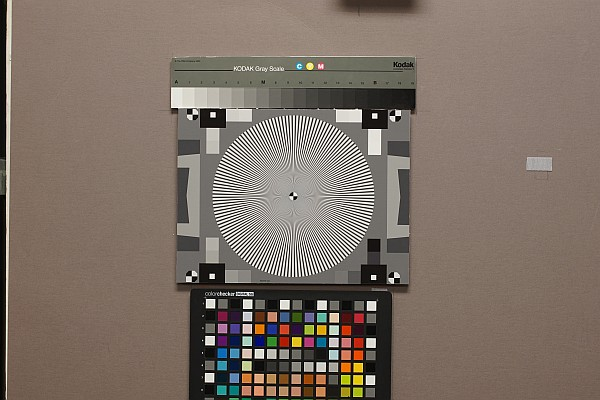Acquire a well-exposed image of the Siemens star in even, glare-free light. Exposures should be reasonably consistent when multiple cameras are tested. The mean pixel level of the linearized image inside the star should be in the range of 0.16 to 0.36. (The optimum has yet to be determined.)

The center of the star should be located close to the center of the image to minimize measurement errors caused by optical distortion (if present). For automatic centering to work properly the image should be oriented so the edges in the center market are nearly vertical and horizontal.

The size of the star in the image should be set so the maximum spatial frequency, corresponding to the minimum radius rmin, is larger than the Nyquist frequency fNyq, and, if possible, no larger than 1.3 fNyq, so sufficient lower frequencies are available for the channel capacity calculation. This means that a 144-cycle star with a 1/20 inner marker should have a diameter of 1400-1750 pixels and a 72-cycle star should have a diameter of 700-875 pixels. For high-quality inkjet printers, the physical diameter of the star should be at least 9 (preferably 12) inches (23 to 30 cm).

Other features may surround the chart, but the average background should be close to neutral gray (18% reflectance) to ensure a good exposure (it is OK to apply exposure compensation if needed). The figure on the right shows a typical star image in a 24-megapixel (4000×6000 pixel) camera.

### Run the Star module

either in Rescharts (interactive; recommended for getting started) or as a fixed, batch-capable module (Star button on the left of the Imatest main window).

In the Star chart settings window, make sure the Calculate information capacity checkbox (near the bottom of the Settings section) is checked. The SNRI settings will be described later. If other settings are correct, press OK.

When OK is pressed the image will be analyzed. Any of several displays can be selected in Rescharts. The table below shows displays that are only available for information capacity measurements.

 Main display Secondary display Description 9.  Information capacity, SNRI SNR (ratio) Signal-to-Noise Ratio (S/N) as a function of spatial frequency for the mean segment and up to 8 individual segments SNR (dB) SNR (dB) as a function of frequency for the mean segment, etc. Signal, Noise Signal, noise, and (S+N)/N (dB) as a function of frequency for the mean segment. Signal, 10X Noise Signal, 10X noise, and (S+N)/N (dB) as a function of frequency for the mean segment. Useful for visualizing low levels of noise NEQ Noise Equivalent Quanta as a function of frequency 10. Difference image (noise-only, etc.) Noise-only (input-noiseless) Display noise-only (with signal removed). This is a remarkable result — possibly the first time that noise has been measured and visualized in the presence of a signal. Loss (input-ideal) Input − Lossless (test chart image). Shows data that has been attenuated. Difficult to interpret. Input image Input image (unmodified) Noiseless image Ideal (noiseless) input image (with MTF loss), derived from Sideal. Ideal image (no MTF loss) “Ideal” image with no MTF loss (represents the original test chart). Noise-only (linear) Noise-only linearized. Typically darker than the gamma-encoded version. Input image (linear) Input image linearized. Typically darker than the gamma-encoded version. 11. 3D Surface plot Displays a 3D surface plot of signal as a function of angle (on the chart) and spatial frequency in Cycles/Pixel. Up to 8 chart cycles are shown (more would be cluttered and difficult to interpret. The image can be rotated. Note that the rectangular (angle × frequency) display area is actually pie-shaped in the chart. A small plot of signal and noise versus frequency and a summary of results is also shown.

### Results

Theww Rescharts displays are specifically designed for information capacity results: 9. Information capacity, SNRI, 10. Input-noiseless Diff, etc., and 11. 3D Surface plot. Here are results from Star run in Rescharts for a raw image (converted to TIFF with dcraw using the 24-bit sRGB preset; gamma ≅ 2.2) for a high quality 24-megapixel Micro Four-Thirds camera.

### Information capacity plot

The plot below shows signal, noise, and (Signal+Noise)/Noise (db)  for the 24-megapixel Micro Four-Thirds Sony A6000, set at ISO 400.Signal, Noise, and Shannon information capacity (3.21 bits/pixel) from a
raw image (converted to TIFF) from a high-quality 24-megapixel Micro Four-Thirds camera @ ISO 400.

 This shows results for an in-camera JPEG the same image capture. The curve has a “bump” that is characteristic of sharpening. Note that the Shannon information capacity is lower than the raw image, even though the JPEG is sharpened. This is happening because the high frequency noise is boosted, along with the signal.   Signal, Noise, and Shannon information capacity (2.92 bits/pixel) from an in-camera JPEG image from a high-quality 24-megapixel Micro Four-Thirds camera @ ISO 400.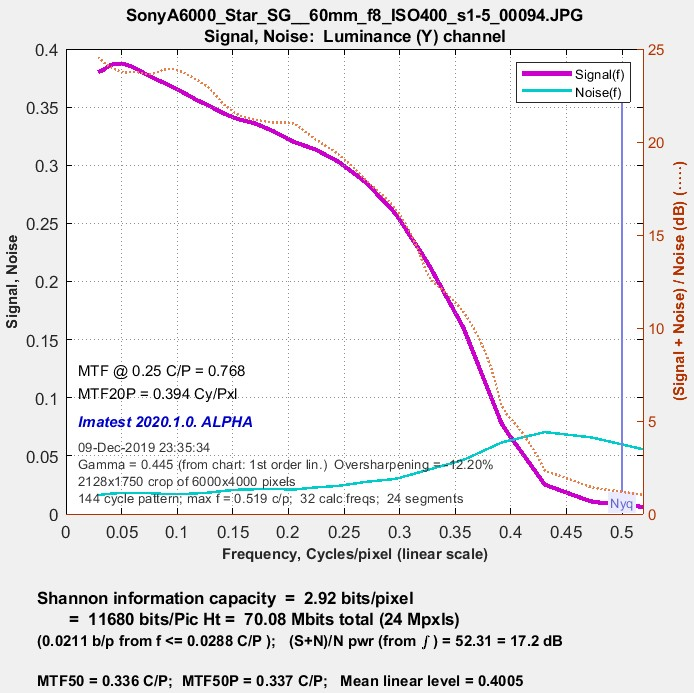### Difference image plot (Input-noiseless, etc.)

The noise-only (input-noiseless difference) plot is of particular interest because images that allow measurement and visualization of noise measured in the presence of a signal (with the sinusoldal star pattern removed) have not been previously available. Because noise is very low, and hence hard to see, at ISO 400, we illustrate noise at ISO 25600 (the maximum for the Micro Four-Thirds camera) for both TIFF from raw and JPEG images. The  Copy image  button on the right copies the image to the clipboard, where it can be pasted into an image editor/viewer or the Image Statistics module for further analysis.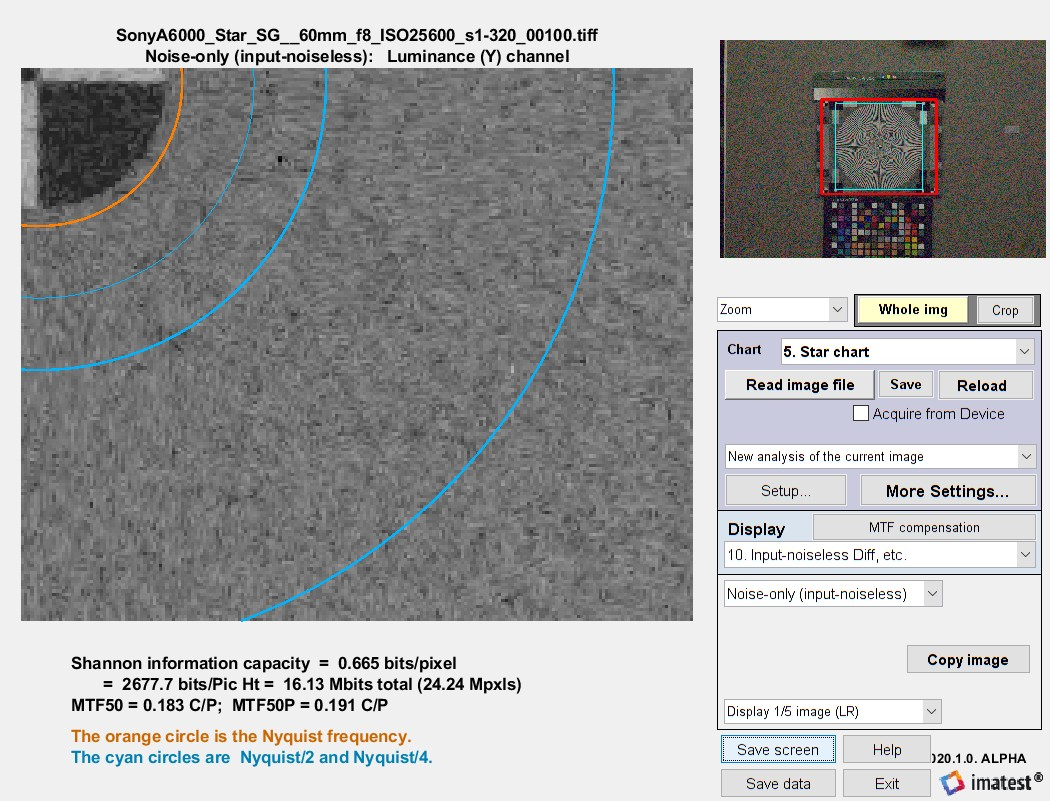Noiseless image for Micro Four-Thirds camera, raw/TIFF image, ISO 25600.

 The image on the right is an in-camera JPEG from the same capture at the above image (ISO 25600). It looks very different from the raw/TIFF image because noise reduction is present. The images below are for raw/TIFF and in-camera JPEG images from the same camera acquired at ISO 400.

### 3D Surface plot

The 3D surface plot allows you to examine small portions of the image in detail.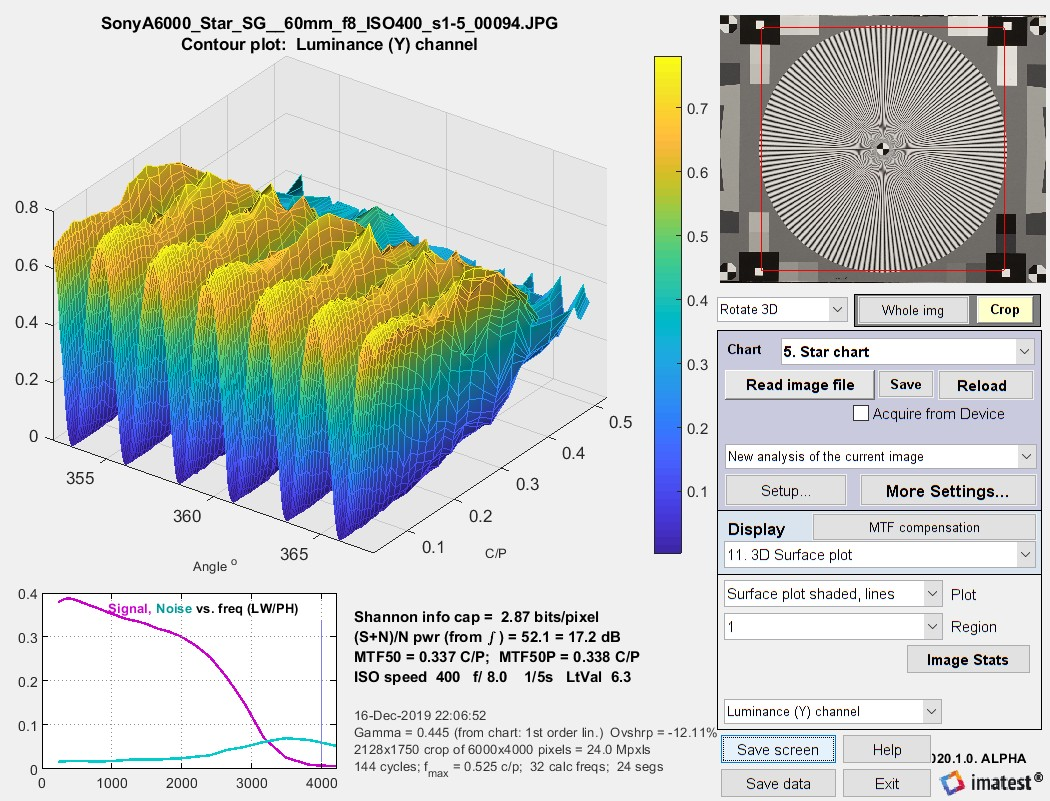3D Surface plot for the high-quality 24 Megapixel Micro Four-Thirds camera analyzed above.

To obtain this display, 3D Surface plot calculation (as well as Calculate information capacity) must be set in the settings window. It shows the signal (for the selected channel) as a function of angle and spatial frequency (in Cycles/Pixel), which is inversely proportional to radius. This plot represents a narrow pie-slice of the original image, with angular detail at high spatial frequencies greatly enlarged.

 A small plot of MTF and noise as a function of spatial frequency is displayed as well as a summary of key results (information capacity, etc.). This plot was motivated by tests on an iPhone 10, where the image appeared to be saturating at low to middle spatial frequencies, but the degree of saturation was difficult to assess by viewing the image. As we can see on the right, saturation is very strong, apparently as a result of some kind of local tone mapping. It is not evident in MTF curves from the star pattern or from the adjacent slanted edges. The iPhone had some Adobe software installed that allowed both raw (DNG) and JPEG software to be captured. We don’t know if this affected the JPEG processing. The image below shows the response of a TIFF file (converted from a DNG raw image from the same iPhone 10). The response is sinusoidal— well-behaved with no amplitude visible distortion. The information capacity is nearly identical to the distorted JPEG image, where several things are happening: random noise is zero where the image is saturated, but noise as defined by $$N(\phi) = S(\phi)-S_{ideal}(\phi)$$ (below), is increased by the amplitude distortion (deviation from the sine function).  The insensitivity of information capacity to image processing, observed in other cases, is a remarkable result. By comparison, MTF50 and MTF50P is very much higher in the highly-processed JPEG image.3D Surface plot from iPhone 10 TIFF from raw (DNG), front view, showing well-behaved sine pattern.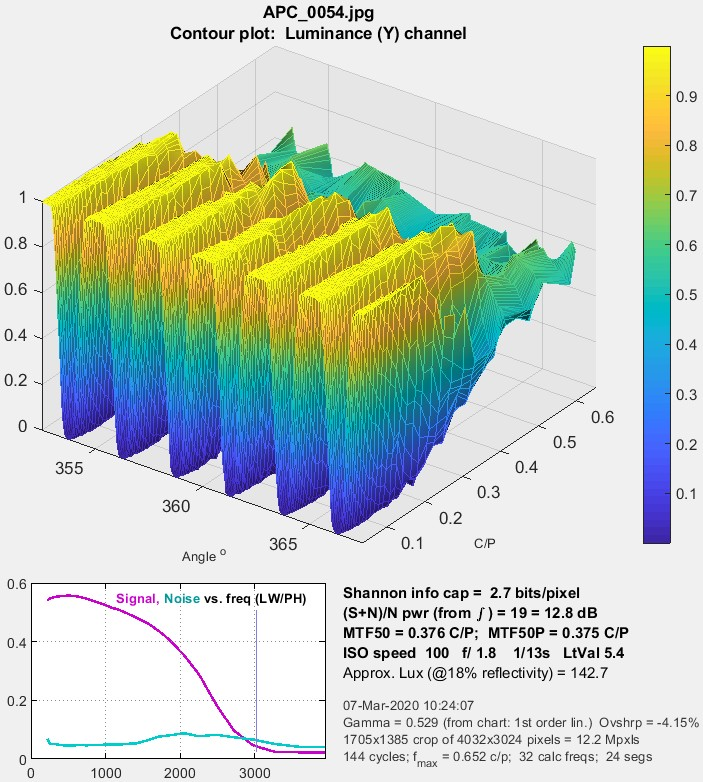3D Surface plot from iPhone 10 JPEG, front view, showing significant clipping (deviation from the expected sine waves).3D Surface plot from iPhone 10 JPEG, rotated (rear) view, showing response rolloff.
Green is for geeks. Do you get excited by a good equation? Were you passionate about your college math classes? Then you’re probably a math geek a member of a misunderstood but highly elite fellowship. The text in green is for you. If you’re normal or mathematically challenged, you may skip these sections. You’ll never know what you missed.

#### Calculating Shannon capacity with Siemens star images

The pixel levels of most interchangeable images (typically encoded in color spaces such as sRGB or Adobe RGB) are gamma-encoded. For these files, pixel level ≅ (sensor illumination)1/gamma, where gamma (typically around 2.2) is the the intended viewing gamma for the color space (display brightness = (pixel level)gamma). To analyze these files they must be linearized by raising the pixel level to the gamma power. RAW files usually don’t need to be linearized (if they were demosaiced without gamma-encoding, i.e., gamma = 1).

The image of the ntotal cycle Siemens star is divided into nr = 32 or 64 radial segments and ns =8 (recommended), 16, or 24 angular segments. Each segment has a period (angular length in radians) P = 2πntotal/ns and contains nk =ntotal / nscycles and kn signal points, each at a known angular location φ, in the range {0, P}.

We assume that the ideal signal in the segment has the form

$$\displaystyle S_{ideal}(\phi) = \sum_{j=1}^{2} a_j \cos \bigl(\frac{2 \pi j n_k \phi}{P} \bigr) + b_j \sin \bigl(\frac{2 \pi j n_k \phi}{P} \bigr)$$

a and b are calculated using the Fourier series coefficient equations, derived from the Wikipedia Fourier Series page, Equation 1.

$$\displaystyle a_j = \frac{2}{P}\int_P S(\phi) \cos \bigl(\frac{2 \pi j n_k \phi}{P} \bigr) d\phi;\quad b_j = \frac{2}{P}\int_P S(\phi) \sin \bigl(\frac{2 \pi j n_k \phi}{P} \bigr) d\phi$$

where S(φ) is the measured signal (actually, signal + noise) in the segment. [Note that although this equation is not in the ISO 12233:2017 standard, it fully satisfies the intent of Appendix F, Step 5 (“A sine curve with the expected frequency is fitted into the measured values by minimizing the square error.”)]

Noise is  $$\displaystyle N(\phi) = S(\phi)-S_{ideal}(\phi)$$

The frequency f in Cycles/Pixel of a segment centered at radius r (in pixels) is $$\displaystyle f = \frac{n_{total}}{2 \pi r}$$.  An interesting consequence of this equation is that it’s easy to locate the Nyquist frequency (0.5 C/P):  $$\displaystyle r = \frac{n_{total}}{\pi}$$ = 45.8 pixels for ntotal = 144 cycles.

A small adjustment (not described here) is made in case f is slightly different from the expected value due to centering errors, optical distortion, or other factors.

Signal power is $$\displaystyle P(f) = \sigma^2(S_{ideal}(f))$$. Noise power is $$\displaystyle N(f) = \sigma^2(N)$$, where σ2 is variance (the square of standard deviation). Note that signal + noise power is $$\displaystyle P(f)+N(f) = \sigma^2(S)$$. [Note: From the context of Shannon: “Communication in the presence of noise”, we assume that N(f) is the noise measured in the presence of signal Sideal(f); not narrow-band noise of frequency f.]

#### Transforming Shannon’s equation from one-dimension to pixels

The full one-dimensional equation for Shannon capacity was presented in Shannon’s second paper in information theory, “Communication in the Presence of Noise,” Proc. IRE, vol. 37, pp. 10-21, Jan. 1949, Eq. (32). This equation cannot be used directly because the pixels under consideration are two-dimensional.

$$\displaystyle C = \int_0^W \log_2 \left( 1 + \frac{S(f)}{N(f)} \right) df = \int_0^W \log_2 \left(\frac{S(f)+N(f)}{N(f)} \right) df$$     [One-dimensional; not used]

This equation has to be converted into two dimensions since pixels (here) have units of area. (They have units of distance for linear measurements like MTF.)

$$\displaystyle C = \int \int_0^W \log_2 \left(\frac{S(f_x,f_y)+N(f_x,f_y)}{N(f_x,f_y)} \right) df_x\: df_y$$

where fx and fy are frequencies in the x and y-directions, respectively. In order to evaluate this integral, we translate x and y into polar coordinates, r and θ.

$$\displaystyle C = \int_0^{2 \pi} \int_0^W \log_2 \left(\frac{S(f_r,f_θ)+N(f_r,f_θ)}{N(f_r,f_θ)} \right) f_r \: df_r\: df_θ$$

Since S and N are only weakly dependent on θ, we can rewrite this equation in one-dimension.

$$\displaystyle C = 2 \pi \int_0^W \log_2 \left(\frac{S(f)+N(f)}{N(f)} \right) f \: df$$

Very geeky: The limiting case for Shannon capacity. Suppose you have an 8-bit pixel. This corresponds to 256 levels (0-255). If you consider the distance of 1 between levels to be the “noise”, then the S/N part of the Shannon equation is log2(1+2562) ≅ 16. The maximum bandwidth where information can be transmitted correctly W— the Nyquist frequency— is 0.5 cycles per pixel. (All signal energy above Nyquist is garbage— disinformation, so to speak.) So C = W log2(1+(S/N)2) = 8 bits per pixel, which is where we started. Sometimes it’s comforting to travel in circles.

### Summary

• Shannon information capacity C has long been used as a measure of the goodness of electronic communication channels. It specifies the maximum rate at which data can be transmitted without error if an appropriate code is used (it took nearly a half-century to find codes that approached the Shannon capacity). Coding is not an issue with imaging.
• C is ordinarily measured in bits per pixel. The total capacity is $$C_{total} = C \times \text{number of pixels}$$.
• The channel must be linearized before C is calculated, i.e., an appropriate gamma correction (signal = pixel levelgamma, where gamma ~= 2) must be applied to obtain correct values of S and N. The value of gamma (close to 2) is determined from runs of any of the Imatest modules that analyze grayscale step charts: StepchartColorcheck., MultichartsMultitestSFRplus, or eSFR ISO.
• We hypothesize that C can be used as a figure of merit for evaluating camera quality, especially for machine vision and Artificial Intelligence cameras. (It doesn’t directly translate to consumer camera appearance because they have to be carefully tuned to reach their potential, i.e., to make pleasing images). It provides a fair basis for comparing cameras, especially when used with images converted from raw with minimal processing.
• Imatest calculates the Shannon capacity C for the Y (luminance; 0.212*R + 0.716*G + 0.072*B) channel of digital images, which approximates the eye’s sensitivity. It also calculates C for the individual R, G, and B channels as well as the Cb and Cr chroma channels (from YCbCr).
• Shannon capacity has not been used to characterize photographic images because it was difficult to calculate and interpret. But now it can be calculated easily, its relationship to photographic image quality is open for study.
• Since C is a new measurement, we will be happy to work with companies or academic institutions who can verify its suitability for Artificial Intelligence systems.
 Note: Because the slanted-edge information capacity measurement used prior to Imatest 2020.1 is inaccurate (correlating poorly with the superior Siemens star measurements) is has been deprecated completely. It has been replaced with a better measurement that is, however, only recommended for use with a Siemens star in the center of the image for calculating the total image information capacity (in units of bits/image).

### Information capacity for the total image

The information capacity we have discussed until now is for a single star, typically located near the center of the image.

The next step after finding the information capacity of a pixel is to find the total capacity, Ctotal, for the camera. Unfortunately, it can’t be reliably obtained by multiplying C by the number of megapixels because lens sharpness (MTF response) tends to be nonuniform, typically decreasing with distance from the image center. To get the total information capacity of the image there are two choices.

1. Use a grid of Siemens star charts, similar to the grid illustrated in the ISO 2014/2017 standard. This is the preferred method when available.
$$C_{total} = \text{mean}(C_{star}) \times \text{megapixels}$$
2. Use a chart with multiple slanted-edges, preferably one of the four Imatest charts with automatic Region of Interest (ROI) detection. This requires capturing a second image.

Slanted-edge method— We have developed a convenient method of calculating Ctotal using an array of slanted-edges. The calculation is based on “star-equivalent information capacity,” CstarEq — the approximate information capacity that would have been calculated from a Siemens star. The procedure sounds complex, but it is easy to preform and quite effective because CstarEq closely tracks the star measurement Cstar (designated as C, above). It does, however, require that a second image be captured.

This method is only recommended for minimally-processed images converted from raw capture because it is strongly affected by sharpening and noise reduction (problematic because sharpness and noise are measured at separate locations for slanted edges). It is not recommended for in-camera JPEG images, and it should not be used as a primary information capacity measurement.

To measure CstarEq, begin by linearizing the image (if required), then measure using low contrast slanted-edges. (4:1 contrast ratio, specified in ISO 12233, is recommended.) Be sure the edge is exposed well, i.e., not saturated. Note that the edge contrast doesn’t directly affect the results.

Measure the pixel levels of the light and dark parts of the slanted-edge region (away from the edge), Pxlt  and Pxdk , and the noise voltages in the two regions, $$N_{lt} = \sigma(Px_{lt})$$ and $$N_{dk} = \sigma(Px_{dk})$$. The mean values of the pixel levels and noise are Pxmean and Nmean, respec­tively. Pxmean should be close to the mean pixel level of the star measurement. Because CstarEq is extremely sensitive to saturation, which reduces the measured values of Ndk or Nlt, it should not be calcu­lated if Pxlt > 0.95 or Pxdk < 0.03.

CstarEq is calculated by Imatest slanted-edge sharpness modules when Secondary readout 1 is set to Secondary readout for star contrast [contrast], where the star contrast (typically 50) should be entered in the [contrast] box. You will need to press the Change readout button in the settings window. The results appear in the Edge & MTF plot.

 For assumed equivalent star contrast Contraststar, the modu­lation (Mich­el­son con­trast) is $$\displaystyle modulation = Px_{mean} \frac {Contrast_{star}-1}{\sqrt{2}(Contrast_{star}+1)}$$ The $$\sqrt{2}$$ factor causes modulation to have units of RMS voltage: useful because modulation2 has units of mean power. The signal power for calculating CstarEq is $$\displaystyle S(f) = (modulation \times MTF(f))^2$$ The noise power of the assumed star is $$\displaystyle N(f) = N^2_{mean}$$ CstarEq is calculated by substituting the above two equations into the Shannon capacity equation. $$\displaystyle C = 2 \pi \int_0^W \log_2 \left(\frac{S(f)+N(f)}{N(f)} \right) f \: df$$ The total camera information capacity is $$\displaystyle C_{total} = \frac{C_{star}(\text{center}) \times \text{mean}(C_{starEq})}{C_{starEq}(\text{center})} \times \text{megapixels}$$  $$\text{mean}(C_{starEq})$$ is the mean of CstarEq taken over a large num­ber of slan­ted-edges distributed over the image

.